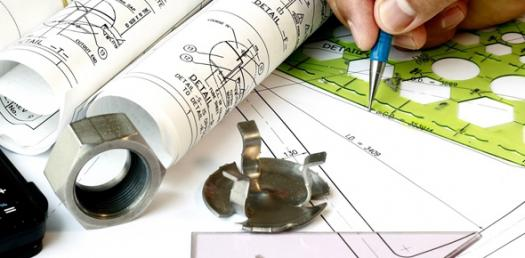# Design And Engineering Vocabulary Test

5 Questions | Total Attempts: 434SettingsDesign and Engineering Vocabulary

• 1.
__________________ is an engineering field that involves manipulating materials on an atomic or molecular level.
• A.

X

• B.

Nanotechnology

• C.

X

• D.

X

• E.

X

• 2.
____________________ is the process of designing new products.
• A.

S

• B.

Invention

• C.

S

• D.

S

• E.

S

• 3.
____________________ is the relationship between how many hours are worked and the quantity of products made in that time period.
• A.

S

• B.

S

• C.

S

• D.

Productivity

• E.

S

• 4.
____________________ are the limits placed on the design and the designer.
• A.

X

• B.

X

• C.

Constraints

• D.

X

• E.

X

• 5.
A _______________ is a working model.
• A.

X

• B.

X

• C.

X

• D.

X

• E.

X

Related TopicsBack to top